# Addition of rational numbers (Math 0)

We’ve already met the idea that rational numbers are what we can make by putting together the building blocks which we get by dividing a single apple into some number of equally-sized pieces; and remember that the building blocks have a special notation, as $$\frac{1}{\text{number}}$$. Recall also that if we take $$5$$ of a certain building block, for instance, we write $$\frac{5}{\text{number}}$$.

It’s clear that if you take one apple and another apple, and put them together, you’ll get two apples. So we should hope that that’s the same if we divided up the apples into pieces first (without removing any of the pieces).

We write $$a+b$$ for “take the rational number $$a$$ and put it next to the rational number $$b$$, and count up what you’ve got”. Because I’m bad at drawing, we’ll pretend apples are just perfect circles. noteThis is actually a design decision: eventually we’ll want to get away from considering apples, and this more abstract representation will be useful.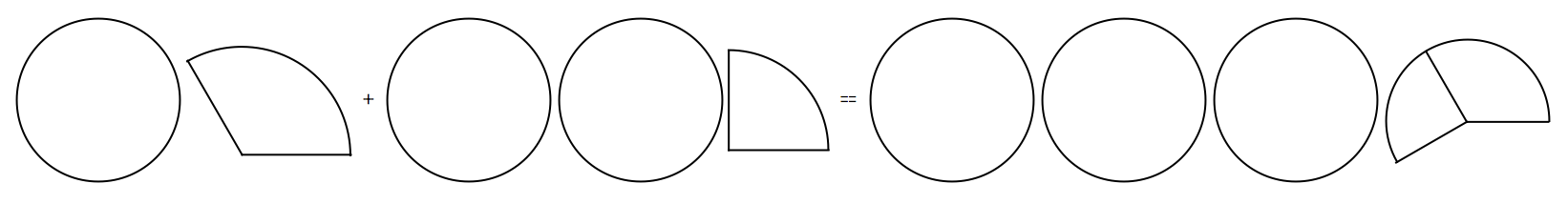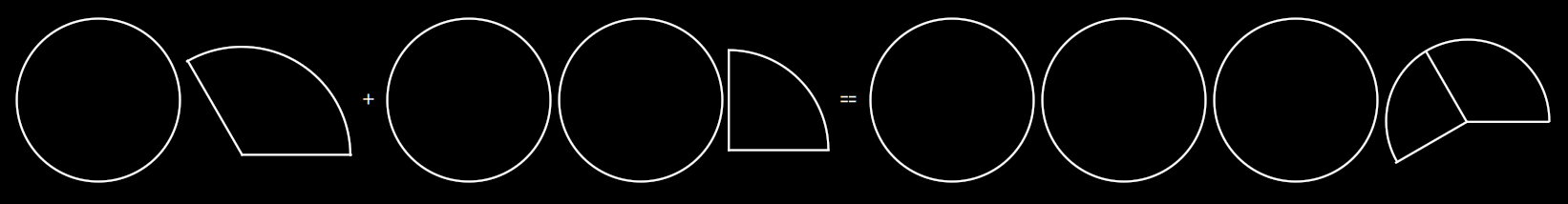For example, we should hope that $$\frac{2}{2} + \frac{3}{3} = 2$$.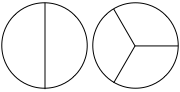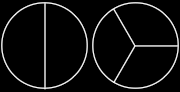What about in cases which aren’t of the form $$\frac{n}{n}$$ for some integer $$n$$? Well, how about $$\frac{5}{3} + \frac{8}{3}$$.If we had five $$\frac{1}{3}$$-blocks, and we put them together with another eight $$\frac{1}{3}$$-blocks, we would hope to have $$5+8=13$$ blocks. So we should hope that $$\frac{5}{3} + \frac{8}{3} = \frac{13}{3}$$.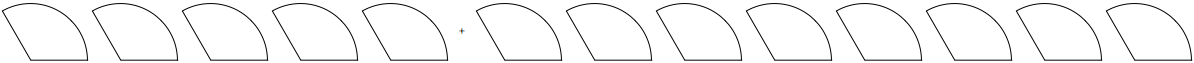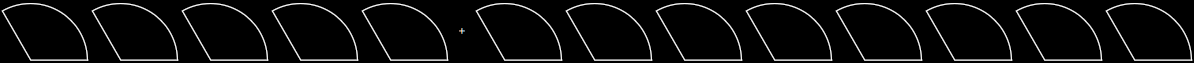And in general, if both our quantities are made up from the same size of building-block (in the above case, the blocks are $$\frac{1}{3}$$-sized), we should just be able to take the two numerators noteRemember, that was the word mathematicians use for the number of blocks we have. and add them together.

Very well. But what if the two quantities are not made up from the same size of building-block? That is, the denominators are different? For example, $$\frac{5}{3} + \frac{5}{4}$$?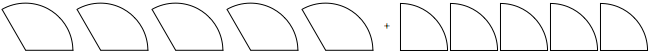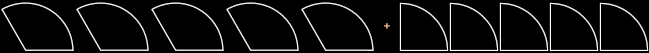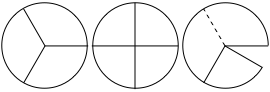Now it’s not so clear. How might we approach this? You should muse on this for thirty seconds before reading on; it will be good for your soul.

The way we do it is to find some smaller block-size, out of which we can make both building-blocks.

# Example

Our example is $$\frac{5}{3} + \frac{5}{4}$$. So we want to make the $$\frac{1}{3}$$ block and the $$\frac{1}{4}$$ block out of some smaller block.

Now, I won’t tell you yet how I got this, but you can check for yourself that $$\frac{1}{12}$$-blocks will make both—$$\frac{1}{3}$$and $$\frac{1}{4}$$-blocks: because three $$\frac{1}{12}$$-blocks together make something the same size as a $$\frac{1}{4}$$-block, and four $$\frac{1}{12}$$-blocks together make something the same size as a $$\frac{1}{3}$$-block.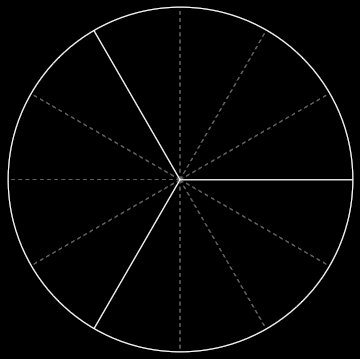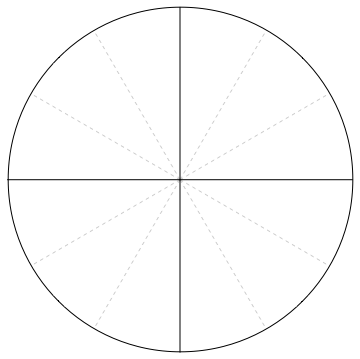Therefore $$\frac{1}{3} = \frac{4}{12}$$: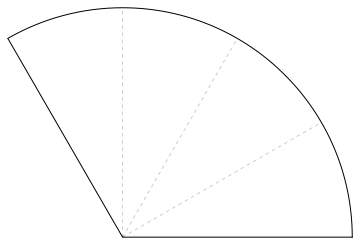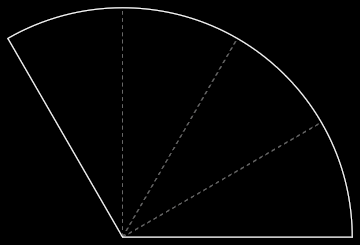And $$\frac{1}{4} = \frac{3}{12}$$: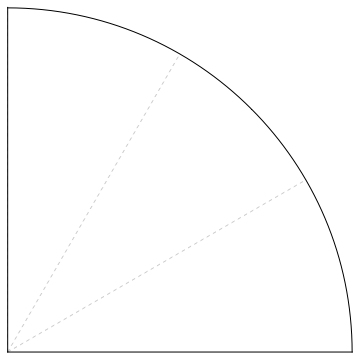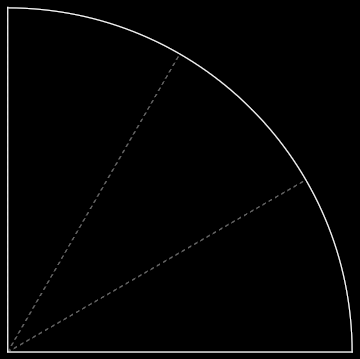Now, if $$\frac{1}{3} = \frac{4}{12}$$ - that is, if one $$\frac{1}{3}$$-piece is the same size as four $$\frac{1}{12}$$-pieces—then it should be the case that five $$\frac{1}{3}$$-pieces come to five lots of four $$\frac{1}{12}$$-pieces. That is, to twenty $$\frac{1}{12}$$-pieces: $$\frac{5}{3} = \frac{20}{12}$$. picture which I haven’t got round to detailing yet

Similarly, $$\frac{5}{4} = \frac{15}{12}$$, because five $$\frac{1}{4}$$-pieces is the same as five lots of three sets of $$\frac{1}{12}$$-pieces, and $$5 \times 3 = 15$$.

Therefore $$\frac{5}{3} + \frac{5}{4}$$ should be just the same as $$\frac{20}{12} + \frac{15}{12}$$, which we know how to calculate! It is $$\frac{35}{12}$$.

# General procedure

The preceding example might suggest a general way for adding two fractions together. (In the process, this should put to rest the existential dread mentioned in the intro to rational numbers, about whether it makes sense to add two rational numbers at all.)

The general procedure is as follows:

1. Find a building-block size such that the building-blocks of both fractions can be made out of that building-block

2. Express each fraction individually in terms of that building-block

3. Add the two together (which we can now do, because they are both made of the same size of building-block).

The interesting part is really the first step.

## Finding a building-block that will neatly make two other differently-sized blocks

Let’s be concrete here: just as above, we showed that $$\frac{1}{12}$$ works for blocks of size $$\frac{1}{3}$$ and $$\frac{1}{4}$$, so we’ll now consider $$\frac{1}{2}$$ and $$\frac{1}{5}$$.

We’re trying to divide up $$\frac{1}{2}$$ into copies of a smaller building-block, such that with copies of that same building block, we can also make $$\frac{1}{5}$$. Here is the key insight that tells us how to do it: let us pretend that we are dividing up the same object, this time a square instead of a circle, into two pieces along one edge and into five pieces along the other edge. How many pieces have we made?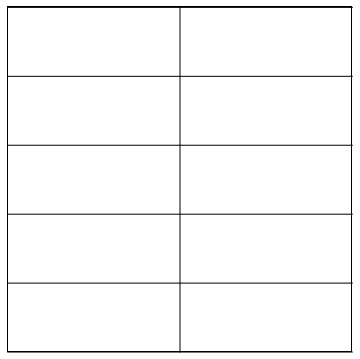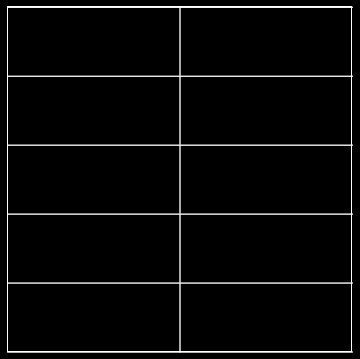There are $$2 \times 5 = 10$$ pieces, each of them the same size (namely the $$\frac{1}{10}$$-block size), and note that as if by magic we’ve just produced a way to subdivide $$\frac{1}{2}$$ into pieces made up of the $$\frac{1}{10}$$-block!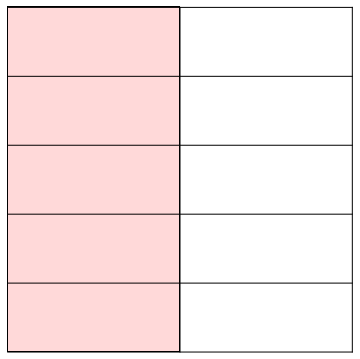And we also have the $$\frac{1}{5}$$ subdivided in the same way!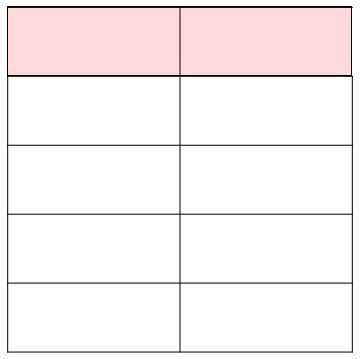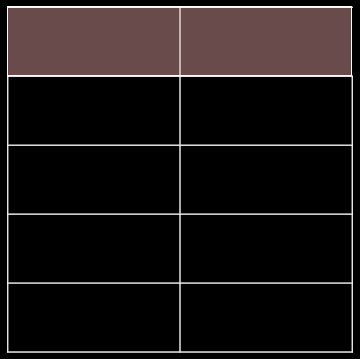This might suggest a general way to do this; you should certainly ponder for thirty seconds before continuing.

find a way to do this without algebra Let’s say we are trying to make both $$\frac{1}{m}$$ and $$\frac{1}{n}$$-sized blocks out of smaller blocks. (So above, $$m$$ is standing for $$2$$ and $$n$$ is standing for $$5$$.)

Then we can do this by splitting both up into $$\frac{1}{m \times n}$$-blocks.

Indeed, $$\frac{1}{n} = \frac{m}{m \times n}$$ (i.e. we can make a $$\frac{1}{n}$$-block out of $$m$$ of the $$\frac{1}{m \times n}$$ tiny-blocks), and $$\frac{1}{m} = \frac{n}{m \times n}$$ (i.e. we can make a $$\frac{1}{m}$$-block out of $$n$$ of the $$\frac{1}{m \times n}$$ tiny-blocks). <div><div>

## Aside: arithmetical rules

The description of the “general way” above has become pretty full of $$\frac{1}{\text{thing}}$$, and refers less and less to the building-blocks we originally considered. The reason for this is that $$\frac{1}{\text{thing}}$$ is an extremely convenient shorthand, and the “general way” can be written as a very simple rule for manipulating that shorthand. Once we have such a convenient rule, we can compute with fractions without having to go through the bother of working out what each fraction really and truly means (in terms of the building-blocks); this saves a lot of time in practice. As an analogy, it’s easier for you to drive a car if, every time you change gears, you don’t have to work out what’s happening inside the gearbox.

The simple rule we have just seen is $$\frac{1}{m} + \frac{1}{n} = \frac{n}{m \times n} + \frac{m}{m \times n}$$$And we’ve already had the rule that $$\frac{a}{m} + \frac{b}{m} = \frac{a+b}{m}$$$ (Remember, if these are not obvious to you, you should think back to what they really mean, in terms of the building-blocks. The first rule is to do with dividing up a big square into little squares, from the previous subsection; the second rule is just taking two collections of building-blocks of the same size and putting them together.) first rule has picture: square divided into n-by-m little squares, with the leftmost vertical column highlighted somehow, indicating that 1/​n = m/​(mn); second rule has picture: $$a$$ things of size 1/​m, next to $$b$$ things of size 1/​m

This is almost every rule we need to add any pair of fractions! We can now add single building blocks of any size together.

To add any pair of rational numbers together—say of building-block size $$\frac{1}{m}$$ and $$\frac{1}{n}$$ respectively—we just need to express them each in terms of the smaller building-block $$\frac{1}{m \times n}$$, and add them together as normal.

Earlier, we considered $$\frac{5}{4} + \frac{5}{3}$$.

A building-block which can make both the $$\frac{1}{4}$$ and $$\frac{1}{3}$$-sized blocks is the $$\frac{1}{12}$$-block, because $$3 \times 4 = 12$$.

Therefore we need to express both our fractions as being made from $$\frac{1}{12}$$-sized blocks.

$$\frac{5}{4}$$ is $$\frac{15}{12}$$, because in each of the five $$\frac{1}{4}$$-blocks, there are three $$\frac{1}{12}$$-blocks, so we have a total of $$5 \times 3$$ blocks of size $$\frac{1}{12}$$.

$$\frac{5}{3}$$ is $$\frac{20}{12}$$, because in each of the five $$\frac{1}{3}$$-blocks, there are four $$\frac{1}{12}$$-blocks, so we have a total of $$5 \times 4 = 20$$ blocks of size $$\frac{1}{12}$$.

Therefore our final answer is $$\frac{15}{12} + \frac{20}{12} = \frac{35}{12}$$. <div><div>

To make this into a general rule: $$\frac{a}{m} + \frac{b}{n} = \frac{a \times n}{m \times n} + \frac{b \times m}{m \times n} = \frac{a \times n + b \times m}{m \times n}$$\$

(Recall the order of operations in the integers: the notation $$a \times n + b \times m$$ means “do $$a \times n$$; then do $$b \times m$$; then finally add them together”. Multiplication comes before addition.)

And that’s it! That’s how we add rational numbers together, and it works even when any or all of $$a, b, m, n$$ are negative. (Remember, though, that $$m$$ and $$n$$ can’t be zero, because it makes no sense to divide something up into no pieces.)

It would be wise now to try the exercises. One learns mathematics through doing, much more than through simply reading; your understanding will be cemented by going through some concrete examples.

Children:

Parents: